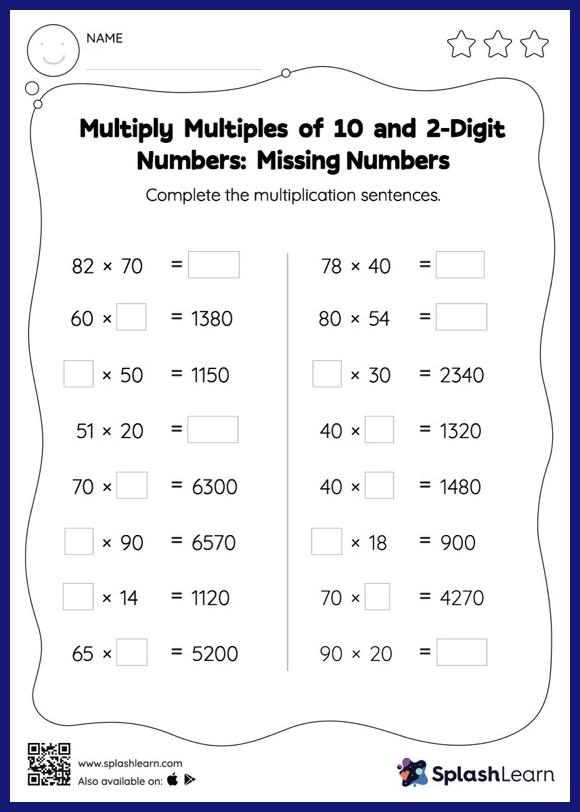# Multiply Multiples of 10 and 2-Digit Numbers: Missing Numbers Worksheet

Home > Multiply Multiples of 10 and 2-Digit Numbers: Missing NumbersThis worksheet invites students to multiply multiples of 10 and 2-digit numbers, which helps them become proficient in multiplication. Students use the pattern of zeros when they are asked to multiply numbers by 10, 100, or their multiples. They apply the same concept to find the missing number in multiply multiples of 10 and 2-digit numbers worksheet. In each problem, the numbers are laid out in the horizontal format. Students should try to use different strategies involving composing and decomposing numbers to solve these problems. This will help them develop flexibility and fluency.